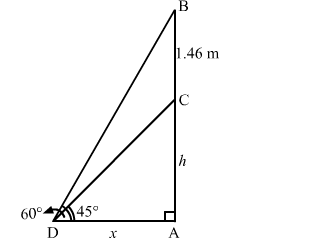# A statue 1.46 m tall, stands on the top of a pedestal.`
Question:

A statue 1.46 m tall, stands on the top of a pedestal. From a point on the ground, the angle of elevation of the top of the statue is 60° and from the same point, the angle of elevation of the top of the pedestal is 45°. Find the height of the pedestal. $[$ Use $\sqrt{3}=1.73]$

Solution:

Let $A C$ be the pedestal and $B C$ be the statue such that $B C=1.46 \mathrm{~m}$.

We have:

$\angle A D C=45^{\circ}$ and $\angle A D B=60^{\circ}$

Let:

$A C=h \mathrm{~m}$ and $A D=x \mathrm{~m}$In the right $\triangle A D C$, we have:

$\frac{A C}{A D}=\tan 45^{\circ}=1$

$\Rightarrow \frac{h}{x}=1$

$\Rightarrow h=x$

Or,

$x=h$

Now, in the right $\triangle A D B$, we have:

$\frac{A B}{A D}=\tan 60^{\circ}=\sqrt{3}$

$\Rightarrow \frac{h+1.46}{x}=\sqrt{3}$

On putting $x=h$ in the above equation, we get:

$\frac{h+1.46}{h}=\sqrt{3}$

$\Rightarrow h+1.46=\sqrt{3} h$

$\Rightarrow h(\sqrt{3}-1)=1.46$

$\Rightarrow h=\frac{1.46}{(\sqrt{3}-1)}=\frac{1.46}{0.73}=2 \mathrm{~m}$

Hence, the height of the pedestal is 2 m.